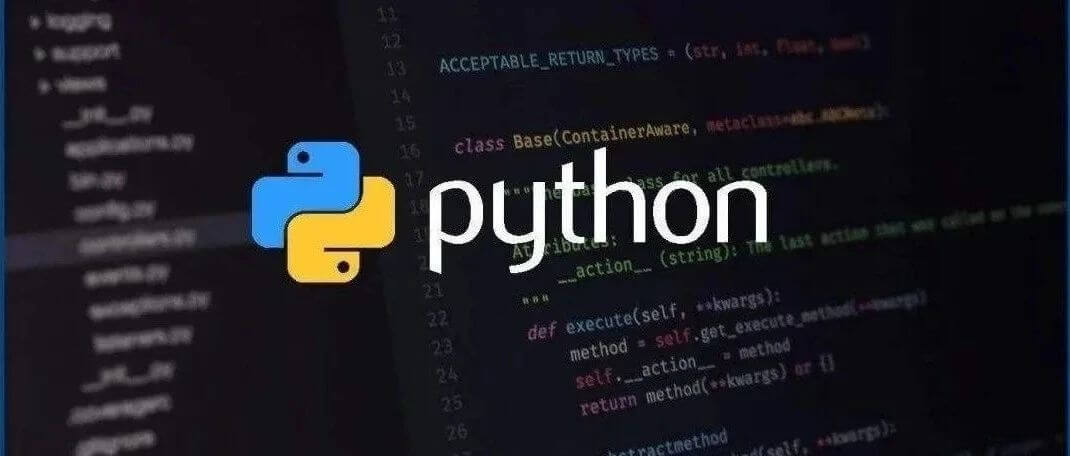Translator: shendeguize@github

## 1、背景

Python 是谷歌主要使用的动态语言，本风格指导列举了使用 Python 编程时应该做和不该做的事项( dos & don’ts )

## 2、Python语言规则

### 2.1 Lint

#### 2.1.1Definition(以下都译为定义)

`pylint`是一个用于在Python代码中发现bug和代码风格问题的工具,，`pylint`查找那些常在非动态语言(例如C或C++)编译器中捕获的问题.由于Python是动态语言,一些警告可能不正确,不过应该非常少有错误警告.

#### 2.1.3 Cons

`pylint`并不完美,为了更好的利用工具,我们有时候需要

a. Write around it(适配上下文风格)

b. 压制一些警告

c. 优化工具

#### 2.1.4 Decision(以下都译为建议)

``dict = 'something awful'  # Bad Idea... pylint: disable=redefined-builtin``

`pylint`警告包含标识名(`empty-docstring`),谷歌专有的警告以`g-`开头.

``pylint --list-msgs``

``pylint --help-msg=C6409``

``def viking_cafe_order(spam, beans, eggs=None):    del beans, eggs  # Unused by vikings.    return spam + spam + spa``

### 2.2 Imports

#### 2.2.4 建议

• `import x`（当`x`是包或模块）
• `from x import y` （当`x`是包前缀，`y`是不带前缀的模块名）
• `from x import  y as z` （当有重复模块名`y``y`过长不利于引用的时候）
• `import y as z` （仅在非常通用的简写的时候使用例如`import numpy as np`

`sound.effects.echo`为例:

``from sound.effects import echo...echo.EchoFilter(input, output, delay=0.7, atten=4)``

### 2.3 包

#### 2.3.3 建议

import示例应该像这样:

Yes:

``# Reference absl.flags in code with the complete name (verbose).# 在代码中使用完整路径调用absl.flagsimport absl.flagsfrom doctor.who import jodieFLAGS = absl.flags.FLAGS``
``# Reference flags in code with just the module name (common).# 在代码中只用包名来调用flagsfrom absl import flagsfrom doctor.who import jodieFLAGS = flags.FLAGS``

No:(假设文件在`doctor/who`中,`jodie.py`也在这里)

``# Unclear what module the author wanted and what will be imported.  The actual# import behavior depends on external factors controlling sys.path.# Which possible jodie module did the author intend to import?# 不清楚作者想要哪个包以及最终import的是哪个包,# 实际的import操作依赖于受到外部参数控制的sys.path# 那么哪一个可能的jodie模块是作者希望import的呢?import jodie``

### 2.4 异常

#### 2.4.4 建议

• 使用`raise MyError('Error message')`或者`raise MyError()`，不要使用两段`raise MyError, 'Error message'`
• 当内置异常类合理的时候,尽量使用内置异常.例如:抛出`ValueError`来表示一个像是违反预设前提(例如传参了一个负数给要求正数的情况)的程序错误发生.

Yes:

``def connect_to_next_port(self, minimum):"""Connects to the next available port.Args:    minimum: A port value greater or equal to 1024.Returns:    The new minimum port.Raises:    ConnectionError: If no available port is found."""if minimum < 1024:    # Note that this raising of ValueError is not mentioned in the doc    # string's "Raises:" section because it is not appropriate to    # guarantee this specific behavioral reaction to API misuse.    # 注意抛出ValueError这件事是不在docstring中的Raises中提及, 因为这样并适合保障对于API误用的特殊反馈    raise ValueError('Minimum port must be at least 1024, not %d.' % (minimum,))port = self._find_next_open_port(minimum)if not port:    raise ConnectionError('Could not connect to service on %d or higher.' % (minimum,))assert port >= minimum, 'Unexpected port %d when minimum was %d.' % (port, minimum)return port``

No:

``def connect_to_next_port(self, minimum):"""Connects to the next available port.Args:    minimum: A port value greater or equal to 1024.Returns:    The new minimum port."""assert minimum >= 1024, 'Minimum port must be at least 1024.'port = self._find_next_open_port(minimum)assert port is not Nonereturn port``
• 库或者包可能会定义各自的异常.当这样做的时候,必须要继承一个已经存在的异常类,异常类的名字应该以`Error`结尾,并且不应该引入重复(`foo.FooError`)

• 永远不要用捕获全部异常的`except:`语句,或者捕获`Exception`或者`StandardError`除非:

Python在这个方面容忍度很高,并且`except:`语句会捕获包括拼写错误,sys.exit(),Ctrl+C终止,单元测试失败和和所有你并没有想到捕获的其他异常.

• 再次抛出这个异常
• 在程序中异常不会继续但是会被记录以及消除(例如通过保护最外层的方式保护线程不会崩溃)的地方创造一个孤立点.
• 最精简`try/except`表达式内部的代码量,`try`代码块里的代码体量越大,月可能会在你不希望抛出异常的代码中抛出异常,进而在这种情况下,`try/except`掩盖了一个真实的异常

• 使用finally来执行代码,这些代码无论是否有异常在`try`代码块被抛出都会被执行.这在清理(即关闭文件)时非常有用.

• 当捕获了异常时,用as而不是逗号分段.

``try:    raise Error()except Error as error:    pass``

### 2.7 列表推导和生成器表达式

#### 2.7.1 定义

List, Dict和Set推导生成式以及生成器表达式提供了一个简明有效的方式来生成容器和迭代器而不需要传统的循环,`map()`,`filter()`或者`lambda表达式`

#### 2.7.4 建议

Yes:

``result = [mapping_expr for value in iterable if filter_expr]result = [{'key': value} for value in iterable          if a_long_filter_expression(value)]result = [complicated_transform(x)          for x in iterable if predicate(x)]descriptive_name = [    transform({'key': key, 'value': value}, color='black')    for key, value in generate_iterable(some_input)    if complicated_condition_is_met(key, value)]result = []for x in range(10):    for y in range(5):        if x * y > 10:            result.append((x, y))return {x: complicated_transform(x)        for x in long_generator_function(parameter)        if x is not None}squares_generator = (x**2 for x in range(10))unique_names = {user.name for user in users if user is not None}eat(jelly_bean for jelly_bean in jelly_beans    if jelly_bean.color == 'black')``

No:

``result = [complicated_transform(          x, some_argument=x+1)          for x in iterable if predicate(x)]result = [(x, y) for x in range(10) for y in range(5) if x * y > 10]return ((x, y, z)        for x in range(5)        for y in range(5)        if x != y        for z in range(5)        if y != z)``

### 2.8 默认迭代器和运算符

#### 建议

Yes:

``for key in adict: ...if key not in adict: ...if obj in alist: ...for line in afile: ...for k, v in adict.items(): ...for k, v in six.iteritems(adict): ...``

No:

``for key in adict.keys(): ...if not adict.has_key(key): ...for line in afile.readlines(): ...for k, v in dict.iteritems(): ...``

### 2.10 Lambda表达式

#### 2.10.1 定义

lambda在一个表达式内定义了匿名函数,而不在语句里.lambda表达式常被用于定义高阶函数(例如`map()``filter()`)使用的回调函数或者操作符.

### 2.11 条件表达式

#### 2.11.4 建议

Yes:

``one_line = 'yes' if predicate(value) else 'no'slightly_split = ('yes' if predicate(value)                  else 'no, nein, nyet')the_longest_ternary_style_that_can_be_done = (    'yes, true, affirmative, confirmed, correct'    if predicate(value)    else 'no, false, negative, nay')``

No:

``bad_line_breaking = ('yes' if predicate(value) else                     'no')portion_too_long = ('yes'                    if some_long_module.some_long_predicate_function(                        really_long_variable_name)                    else 'no, false, negative, nay')``

### 2.12 默认参数值

#### 2.12.4 建议

Yes:

``def foo(a, b=None):    if b is None:        b = []def foo(a, b: Optional[Sequence] = None):    if b is None:        b = []def foo(a, b: Sequence = ()):  # Empty tuple OK since tuples are immutable 空元组是也不可变的    ...``

No:

``def foo(a, b=[]):    ...def foo(a, b=time.time()):  # The time the module was loaded??? 模块被加载的时间???    ...def foo(a, b=FLAGS.my_thing):  # sys.argv has not yet been parsed... sys.argv还未被解析    ...def foo(a, b: Mapping = {}):  # Could still get passed to unchecked code 仍可传入未检查的代码(此处翻译可能有误)    ...``

### 2.13 属性

#### 2.13.4 建议

Yes:

``class Square(object):    """A square with two properties: a writable area and a read-only perimeter.    To use:    >>> sq = Square(3)    >>> sq.area    9    >>> sq.perimeter    12    >>> sq.area = 16    >>> sq.side    4    >>> sq.perimeter    16    """    def __init__(self, side):        self.side = side    @property    def area(self):        """Area of the square."""        return self._get_area()    @area.setter    def area(self, area):        return self._set_area(area)    def _get_area(self):        """Indirect accessor to calculate the 'area' property."""        return self.side ** 2    def _set_area(self, area):        """Indirect setter to set the 'area' property."""        self.side = math.sqrt(area)    @property    def perimeter(self):        return self.side * 4``

### 2.14 True/False表达式

#### 建议

• 使用`if foo is None`(或者`if foo is not None`)来检查`None`.例如在检查一个默认值是`None`的变量或者参数是否被赋予了其他值的时候,被赋予的其他值的布尔值可能为False.
• 不要用`==`来和布尔值为`False`的变量比较,使用`if not x`,如果需要区别`False``None`,那么使用链式的表达式如`if not x and x is not None`
• 对于序列(如字符串,列表,元组),利用空序列为`False`的事实,故而相应地使用`if seq:``if not seq:`而非`if len(seq)``if not len(seq):`.
• 在处理整数时,隐式的False可能会引入更多风险(例如意外地将`None`和0进行了相同的处理)你可以用一个已知是整形(并且不是`len()`的结果)的值和整数0比较.

Yes:

``if not users:    print('no users')if foo == 0:    self.handle_zero()if i % 10 == 0:    self.handle_multiple_of_ten()def f(x=None):    if x is None:        x = []``

No:

``if len(users) == 0:    print('no users')if foo is not None and not foo:    self.handle_zero()if not i % 10:    self.handle_multiple_of_ten()def f(x=None):    x = x or []``

### 2.15 弃用的语言特性

#### 2.15.2 建议

Yes:

``words = foo.split(':')[x for x in my_list if x == 5]map(math.sqrt, data)    # Ok. No inlined lambda expression. 可以,没有行内的lambda表达式fn(*args, **kwargs)``

No:

``words = string.split(foo, ':')map(lambda x: x, filter(lambda x: x == 5, my_list))apply(fn, args, kwargs)``

### 2.16 词法作用域

#### 2.16.1 定义

``def get_adder(summand1):    """Returns a function that adds numbers to a given number."""    def adder(summand2):        return summand1 + summand2    return adder``

#### 2.16.3 Cons

``i = 4def foo(x):    def bar():        print(i, end='')    # ...    # A bunch of code here    # ...    for i in x:  # Ah, i *is* local to foo, so this is what bar sees i对于foo来说是局部变量,所以在这里就是bar函数所获取的值        print(i, end='')    bar()``

### 2.17 函数和方法装饰器

#### 2.17.1 定义

``class C(object):    @my_decorator    def method(self):        # method body ...``

``class C(object):    def method(self):        # method body ...    method = my_decorator(method)``

### 2.19 过于强大的特性

#### 2.19.1 定义

Python是一种非常灵活的语言并且提供了很多新奇的特性,诸如定制元类,访问字节码,动态编译,动态继承,对象父类重定义,import hacks,反射(例如一些对于`getattr()`的应用),系统内置的修改等等.

### 2.20 新版本Python: Python3 和从`__future__`import

Python3已经可用了(译者:目前Python2已经不受支持了),尽管不是每个项目都准备好使用Python3,所有的代码应该兼容Python3并且在可能的情况下在Python3的环境下测试.

#### 2.20.1 定义

Python3是Python的重大改变,尽管现有代码通常是Python2.7写成的,但可以做一些简单的事情来让代码更加明确地表达其意图,从而可以让代码更好地在Python3下运行而不用调整.

#### 2.20.4 建议

from future imports

``from __future__ import absolute_importfrom __future__ import divisionfrom __future__ import print_function``

The six, future, or past libraries

### 2.21 带有类型注释的代码

#### 2.21.1 定义

``def func(a: int) -> List[int]:``

``a = SomeFunc()  # type: SomeType``

## 3、Python代码风格规范

### 3.2 行长度

• 长import
• 注释中的：URL,路径,flags等
• 不包含空格不方便分行的模块级别的长字符串常量
• pylint的diable注释使用(如`# pylint: disable=invalid-name`)

Yes:

``foo_bar(self, width, height, color='black', design=None, x='foo',        emphasis=None, highlight=0)if (width == 0 and height == 0 and    color == 'red' and emphasis == 'strong'):``

``x = ('This will build a very long long '     'long long long long long long string')``

Yes:

``# See details at# http://www.example.com/us/developer/documentation/api/content/v2.0/csv_file_name_extension_full_specification.html``

No:

``# See details at# http://www.example.com/us/developer/documentation/api/content/\# v2.0/csv_file_name_extension_full_specification.html``

Yes:

``with very_long_first_expression_function() as spam, \     very_long_second_expression_function() as beans, \     third_thing() as eggs:    place_order(eggs, beans, spam, beans)with very_long_first_expression_function() as spam:    with very_long_second_expression_function() as beans:        place_order(beans, spam)``

No:

``with VeryLongFirstExpressionFunction() as spam, \     VeryLongSecondExpressionFunction() as beans:    PlaceOrder(eggs, beans, spam, beans)``

### 3.3 括号

Yes:

``if foo:    bar()while x:    x = bar()if x and y:    bar()if not x:    bar()# For a 1 item tuple the ()s are more visually obvious than the comma.onesie = (foo,)return fooreturn spam, beansreturn (spam, beans)for (x, y) in dict.items(): ...``

No:

``if (x):    bar()if not(x):    bar()return (foo)``

### 3.4 缩进

Yes:

``# Aligned with opening delimiter# 和opening delimiter对齐(译者理解是分隔符的入口,例如三种括号,字符串引号等)foo = long_function_name(var_one, var_two,                         var_three, var_four)meal = (spam,        beans)# Aligned with opening delimiter in a dictionaryfoo = {    long_dictionary_key: value1 +                         value2,    ...}# 4-space hanging indent; nothing on first line# 缩进4个空格,首行括号后无内容foo = long_function_name(    var_one, var_two, var_three,    var_four)meal = (    spam,    beans)# 4-space hanging indent in a dictionaryfoo = {    long_dictionary_key:        long_dictionary_value,    ...}``

No:

``# Stuff on first line forbidden# 首行不允许有内容foo = long_function_name(var_one, var_two,    var_three, var_four)meal = (spam,    beans)# 2-space hanging indent forbiddenfoo = long_function_name(  var_one, var_two, var_three,  var_four)# No hanging indent in a dictionaryfoo = {    long_dictionary_key:    long_dictionary_value,    ...}``

#### 3.4.1 关于尾后逗号

Yes:

``golomb3 = [0, 1, 3]golomb4 = [    0,    1,    4,    6,]``

No:

``golomb4 = [    0,    1,    4,    6]``

### 3.6 空格

Yes:

``spam(ham, {eggs: 2}, [])``

No:

``spam( ham[ 1 ], { eggs: 2 }, [ ] )``

Yes:

``if x == 4:    print(x, y)x, y = y, x``

No:

``if x == 4 :    print(x , y)x , y = y , x``

Yes:

``spam(1)dict['key'] = list[index]``

No:

``spam (1)dict ['key'] = list [index]``

Yes:

``x == 1``

No:

``x<1``

Yes:

``def complex(real, imag=0.0): return Magic(r=real, i=imag)def complex(real, imag: float = 0.0): return Magic(r=real, i=imag)``

No:

``def complex(real, imag = 0.0): return Magic(r = real, i = imag)def complex(real, imag: float=0.0): return Magic(r = real, i = imag)``

Yes:

``foo = 1000  # commentlong_name = 2  # comment that should not be aligneddictionary = {    'foo': 1,    'long_name': 2,}``

No:

``foo       = 1000  # commentlong_name = 2     # comment that should not be aligneddictionary = {    'foo'      : 1,    'long_name': 2,}``

### 3.8 注释和文档字符串

#### 3.8.1 文档字符串

Python使用文档字符串来为代码生成文档.文档字符串是包,模块,类或函数的首个语句.这些字符串能够自动被`__doc__`成员方法提取并且被`pydoc`使用.(尝试在你的模块上运行`pydoc`来看看具体是什么).文档字符串使用三重双引号`"""`(根据PEP-257).文档字符串应该这样组织:一行总结(或整个文档字符串只有一行)并以句号,问好或感叹号结尾.随后是一行空行,随后是文档字符串,并与第一行的首个引号位置相对齐.更多具体格式规范如下.

#### 3.8.2 模块

``"""A one line summary of the module or program, terminated by a period.Leave one blank line.  The rest of this docstring should contain anoverall description of the module or program.  Optionally, it may alsocontain a brief description of exported classes and functions and/or usageexamples.  Typical usage example:  foo = ClassFoo()  bar = foo.FunctionBar()"""``

#### 3.8.3 函数和方法

• 外部不可见
• 非常短
• 简明

Args:

Returns:(或对于生成器是Yields:)

Raises:

``def fetch_bigtable_rows(big_table, keys, other_silly_variable=None):    """Fetches rows from a Bigtable.    Retrieves rows pertaining to the given keys from the Table instance    represented by big_table.  Silly things may happen if    other_silly_variable is not None.    Args:        big_table: An open Bigtable Table instance.        keys: A sequence of strings representing the key of each table row            to fetch.        other_silly_variable: Another optional variable, that has a much            longer name than the other args, and which does nothing.    Returns:        A dict mapping keys to the corresponding table row data        fetched. Each row is represented as a tuple of strings. For        example:        {'Serak': ('Rigel VII', 'Preparer'),         'Zim': ('Irk', 'Invader'),         'Lrrr': ('Omicron Persei 8', 'Emperor')}        If a key from the keys argument is missing from the dictionary,        then that row was not found in the table.    Raises:        IOError: An error occurred accessing the bigtable.Table object.    """``

#### 3.8.4 类

``class SampleClass(object):    """Summary of class here.    Longer class information....    Longer class information....    Attributes:        likes_spam: A boolean indicating if we like SPAM or not.        eggs: An integer count of the eggs we have laid.    """    def __init__(self, likes_spam=False):        """Inits SampleClass with blah."""        self.likes_spam = likes_spam        self.eggs = 0    def public_method(self):        """Performs operation blah."""``

#### 3.8.5 块注释和行注释

``# We use a weighted dictionary search to find out where i is in# the array.  We extrapolate position based on the largest num# in the array and the array size and then do binary search to# get the exact number.if i & (i-1) == 0:  # True if i is 0 or a power of 2.``

### 3.9 类

Yes:

``class SampleClass(object):    passclass OuterClass(object):    class InnerClass(object):        passclass ChildClass(ParentClass):    """Explicitly inherits from another class already."""``

No:

``class SampleClass:    passclass OuterClass:    class InnerClass:        pass``

`object`类继承保证了属性能够在Python2正确运行并且保护代码在Python3下出现潜在的不兼容.这样也定义了object包括`__new__`,`__init__`,`__delattr__`,`__getattribute__`,`__setattr__`,`__hash__`,`__repr__`,和`__str__`等默认特殊方法的实现.

### 3.10 字符串

Yes:

``x = a + bx = '%s, %s!' % (imperative, expletive)x = '{}, {}'.format(first, second)x = 'name: %s; score: %d' % (name, n)x = 'name: {}; score: {}'.format(name, n)x = f'name: {name}; score: {n}'  # Python 3.6+``

No:

``employee_table = '<table>'for last_name, first_name in employee_list:    employee_table += '<tr><td>%s, %s</td></tr>' % (last_name, first_name)employee_table += '</table>'``

Yes:

``items = ['<table>']for last_name, first_name in employee_list:    items.append('<tr><td>%s, %s</td></tr>' % (last_name, first_name))items.append('</table>')employee_table = ''.join(items)``

No:

``employee_table = '<table>'for last_name, first_name in employee_list:    employee_table += '<tr><td>%s, %s</td></tr>' % (last_name, first_name)employee_table += '</table>'``

Yes:

``Python('Why are you hiding your eyes?')Gollum("I'm scared of lint errors.")Narrator('"Good!" thought a happy Python reviewer.')``

No:

``Python("Why are you hiding your eyes?")Gollum('The lint. It burns. It burns us.')Gollum("Always the great lint. Watching. Watching.")``

No:

``long_string = """This is pretty ugly.Don't do this."""``

Yes:

``long_string = """This is fine if your use case can accept    extraneous leading spaces."""long_string = ("And this is fine if you can not accept\n" +               "extraneous leading spaces.")long_string = ("And this too is fine if you can not accept\n"               "extraneous leading spaces.")import textwraplong_string = textwrap.dedent("""\    This is also fine, because textwrap.dedent()    will collapse common leading spaces in each line.""")``

### 3.11 文件和socket

• 他们可能会消耗有限的系统资源,例如文件描述符.如果在使用没有即使归还系统,处理很多这样对象的代码可能会浪费掉很多不应浪费的资源.
• 保持一个文件可能会阻止其他操作诸如移动或删除.
• 被程序共享的文件和socket可能会无意中在逻辑上已被关闭的情况下仍被读写.如果实际上已经关闭,试图读写的操作会抛出异常,这样就可以立即发现问题.

• 不能保证何时会真正将文件对象销毁.不同的Python解释器使用的内存管理技术不同,例如延时垃圾处理可能会让对象的生命周期被无限期延长.
• 可能导致意料之外地对文件对象的引用,例如在全局变量或者异常回溯中,可能会让文件对象比预计的生命周期更长.

``with open("hello.txt") as hello_file:    for line in hello_file:        print(line)``

``import contextlibwith contextlib.closing(urllib.urlopen("http://www.python.org/")) as front_page:    for line in front_page:        print(line)``

### 3.12  TODO注释

`TODO`注释以全部大写的字符串`TODO`开头,并带有写入括号内的姓名,email地址,或其他可以标识负责人或者包含关于问题最佳描述的issue.随后是这里做什么的说明.

``# TODO(kl@gmail.com): Use a "*" here for string repetition.# TODO(Zeke) Change this to use relations.``

### 3.13 import格式

imports应该在不同行.例如:

Yes:

``import osimport sys``

No:

``import os, sys``

import应集中放在文件顶部,在模块注释和docstring后面,模块globals和常量前面.应按照从最通用到最不通用的顺序排列分组:

1. Python未来版本import语句,例如:

``from __future__ import absolute_importfrom __future__ import divisionfrom __future__ import print_function``

更多信息参看上文

2. Python标准基础库import,例如:

``import sys``
3. 第三方库或包的import,例如:

``import tensorflow as tf``
4. 代码库内子包import,例如:

``from otherproject.ai import mind``
5. 此条已弃用:和当前文件是同一顶级子包专用的import,例如:

``from myproject.backend.hgwells import time_machine``

在旧版本的谷歌Python代码风格指南中实际上是这样做的.但是现在不再需要了.**新的代码风格不再受此困扰.**简单的将专用的子包import和其他子包import同一对待即可.

``import collectionsimport queueimport sysfrom absl import appfrom absl import flagsimport bs4import cryptographyimport tensorflow as tffrom book.genres import scififrom myproject.backend.hgwells import time_machinefrom myproject.backend.state_machine import main_loopfrom otherproject.ai import bodyfrom otherproject.ai import mindfrom otherproject.ai import soul# Older style code may have these imports down here instead:# 旧版本代码风格可能会采用下述import方式# from myproject.backend.hgwells import time_machine# from myproject.backend.state_machine import main_loop``

### 3.14 语句

Yes:

``if foo: bar(foo)``

No:

``if foo: bar(foo)else:   baz(foo)try:               bar(foo)except ValueError: baz(foo)try:    bar(foo)except ValueError: baz(foo)``

### 3.16 命名

`module_name`,`package_name`,`ClassName`,`method_name`,`ExceptionName`,`function_name`,`GLOBAL_CONSTANT_NAME`,`global_var_name`,`instance_var_name`,`function_parameter_name`,`local_var_name`.

#### 3.16.1 要避免的名字：

• 单字符名字,除非是计数或迭代元素,e可以作为Exception捕获识别名来使用..
• `-`横线,不应出现在任何包名或模块名内
• `__double_leading_and_trailing_underscore__`首尾都双下划线的名字,这种名字是python的内置保留名字

#### 3.16.4 命名约定

• internal表示仅模块内可用、或者类内保护的或者私有的
• 单下划线(`_`)开头表示是被保护的(`from module import *`不会import).双下划线(`__`也就是”dunder”)开头的实例变量或者方法表示类内私有(使用命名修饰).我们不鼓励使用,因为这会对可读性和可测试性有削弱二期`并非真正`的私有.
• 相关的类和顶级函数放在同一个模块内,不必像是Java一样要一个类放在一个模块里.
• 对类名使用大写字母(如CapWords)开头的单词,命名,模块名应该使用小写加下划线的方式.尽管有一些旧的模块命名方式是大写字母的(如CapWords.py),现在不鼓励这样做了,因为在模块刚好是从某个类命名出发的时候可能会令人迷惑(例如是选择`import StringIO`还是`from StringIO import StringIO`?)
• unittest方法中可能是`test`开头来分割名字的组成部分,即使这些组成部分是使用大写字母驼峰式的.这种方式是可以的：`test<MethodUnderTest>_<state>`例如`testPop_EmptyStack`,对于命名测试方法没有明确的正确方法.

#### 3.16.4 Guido的指导建议

`lower_with_under`

`CapWords` `_CapWords`

### 3.17 Main

``def main():    ...if __name__ == '__main__':    main()``

### 3.19 类型注释

#### 3.19.1 基本规则

• 熟悉PEP-484
• 在方法中,只在必要时给`self`或者`cls`增加合适的类型信息.例如`@classmethod def create(cls: Type[T]) -> T: return cls()`
• 如果其他变量或返回类型不定,使用`Any`
• 不需要注释每个函数
• 至少需要注明公共接口
• 使用类型检查来在安全性和声明清晰性以及灵活性之间平衡
• 标注容易因类型相关而抛出异常的代码(previous bugs or complexity,此处译者认为是与上一条一致,平衡安全性和复杂性)
• 标注难理解的代码
• 标注类型稳定的代码,成熟稳定的代码可以都进行标注而不会影响其灵活性

#### 3.19.2 分行

``def my_method(self,              first_var: int,              second_var: Foo,              third_var: Optional[Bar]) -> int:  ...``

``def my_method(self, first_var: int) -> int:  ...``

``def my_method(    self, first_var: int) -> Tuple[MyLongType1, MyLongType1]:  ...``

``def my_method(    self, other_arg: Optional[MyLongType]) -> Dict[OtherLongType, MyLongType]:  ...``

pylint允许您将右括号移动到新行并与左括号对齐,但这不太容易理解.

No:

``def my_method(self,              other_arg: Optional[MyLongType]             ) -> Dict[OtherLongType, MyLongType]:  ...``

``def my_method(    self,    first_var: Tuple[List[MyLongType1],                     List[MyLongType2]],    second_var: List[Dict[        MyLongType3, MyLongType4]]) -> None:  ...``

Yes:

``def my_function(    long_variable_name:        long_module_name.LongTypeName,) -> None:  ...``

No:

``def my_function(    long_variable_name: long_module_name.        LongTypeName,) -> None:  ...``

#### 3.19.3 前置声明

``class MyClass(object):  def __init__(self,               stack: List["MyClass"]) -> None:``

#### 3.19.4 默认值

Yes:

``def func(a: int = 0) -> int:  ...``

No:

``def func(a:int=0) -> int:  ...``

#### 3.19.5 NoneType

Yes:

``def func(a: Optional[Text], b: Optional[Text] = None) -> Text:  ...def multiple_nullable_union(a: Union[None, Text, int]) -> Text  ...``

No:

``def nullable_union(a: Union[None, Text]) -> Text:  ...def implicit_optional(a: Text = None) -> Text:  ...``

#### 3.19.6 类型别名

``_ShortName = module_with_long_name.TypeWithLongNameComplexMap = Mapping[Text, List[Tuple[int, int]]]``

#### 3.19.7 忽略类型检查

`pytype`对于明确的报错有关闭选项(类似于lint):

``# pytype: disable=attribute-error``

#### 3.19.8 对变量注释类型

``a = SomeUndecoratedFunction()  # type: Foo``

``a: Foo = SomeUndecoratedFunction()``

#### 3.19.9 元组和列表

``a = [1, 2, 3]  # type: List[int]b = (1, 2, 3)  # type: Tuple[int, ...]c = (1, "2", 3.5)  # type: Tuple[int, Text, float]``

#### 3.19.10 TypeVars

Python是有泛型的,工厂函数`TypeVar`是通用的使用方式.

``from typing import List, TypeVarT = TypeVar("T")...def next(l: List[T]) -> T:  return l.pop()``

TypeVar可以约束类型:

``AddableType = TypeVar("AddableType", int, float, Text)def add(a: AddableType, b: AddableType) -> AddableType:    return a + b``

`typing`模块预定义好的类型变量是`AnyStr`,用于针对字符串可以是`bytes`也可为`unicode`并且保持一致的多个类型注释.

``from typing import AnyStrdef check_length(x: AnyStr) -> AnyStr:  if len(x) <= 42:    return x  raise ValueError()``

#### 3.19.11 字符串类型

No:

``def py2_code(x: str) -> unicode:  ...``

Yes:

``def deals_with_binary_data(x: bytes) -> bytes:  ...``

``from typing import Text...def py2_compatible(x: Text) -> Text:  ...def py3_only(x: str) -> str:  ...``

``from typing import Text, Union...def py2_compatible(x: Union[bytes, Text]) -> Union[bytes, Text]:  ...def py3_only(x: Union[bytes, str]) -> Union[bytes, str]:  ...``

#### 3.19.12 typing的import

``from typing import Any, Dict, Optional``

``from typing import Any as AnyType``

#### 3.19.13 条件import

• 条件import的类型应被视为字符串引用,以和Python3.6兼容(在Python3.6中,注释表达式实际上被赋值的).
• 只有单独用于类型注释的实例才能在这里定义,包括了别名.否则将会报运行错误因为在运行时这些模块不会被引用.
• 代码块应该紧跟在正常import后面.
• 在类型import后不应有空行
• 按照正常import顺序对这一块代码进行排序
``import typingif typing.TYPE_CHECKING:    import sketchdef f(x: "sketch.Sketch"): ...``

#### 3.19.14 循环依赖

``from typing import Anysome_mod = Any  # some_mod.py imports this module....def my_method(self, var: some_mod.SomeType) -> None:  ...``

#### 3.19.15  泛型

``def get_names(employee_ids: List[int]) -> Dict[int, Any]:  ...``
``# These are both interpreted as get_names(employee_ids: List[Any]) -> Dict[Any, Any]def get_names(employee_ids: list) -> Dict:  ...def get_names(employee_ids: List) -> Dict:  ...``

``def get_names(employee_ids: List[Any]) -> Dict[Any, Text]:"""Returns a mapping from employee ID to employee name for given IDs."""``
``T = TypeVar('T')def get_names(employee_ids: List[T]) -> Dict[T, Text]:"""Returns a mapping from employee ID to employee name for given IDs."""``

## 4、最后的话

¥1¥5¥10¥20¥50¥100¥200 自定义

​Python实用宝典 ( pythondict.com )